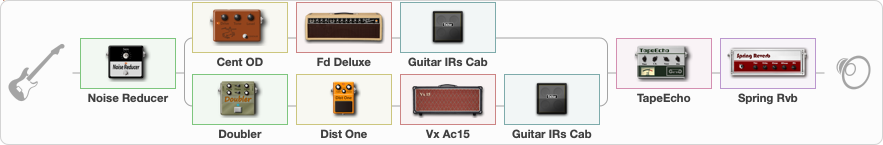# belloved

Discussion in 'ToneLib-GFX presets' started by belloved, May 15, 2020.

1. belloved

Preset name: Splitted Crunch

Effects chain:Effect: "Noise Reducer" (Dynamics / Filter), active - "yes"
{
"Sens" = 61
"Mode" = Soft
}

Effect: "Splitter" (Dynamics / Filter), active - "yes"
{
"A-Bypass" = Off
"A-Pan" = -66
"A-Level" = 55
"B-Bypass" = Off
"B-Pan" = 31
"B-Level" = 81

'A' branch:
{

Effect: "Cent OD" (Overdrive / Distortion), active - "yes"
{
"Drive" = 28
"Tone" = 61
"Level" = 52
}

Effect: "Fd Deluxe" (Amp simulators), active - "yes"
{
"Gain" = 61
"Bass" = 58
"Middle" = 57
"Treble" = 66
"Presence" = 69
"Master" = 66
"Level (dB)" = 0
}

Effect: "Guitar IRs Cab" (Cabinets), active - "yes"
{
"Model" = Bogner Ecstasy (4x12")
"Mic Position" = Center
"Mic Distance" = Middle
"Low Cut (Hz)" = 60
"Hi Cut (kHz)" = 20.0
"Mix" = 100
"Level (dB)" = 0
}
}
'B' branch:
{

Effect: "Doubler" (Dynamics / Filter), active - "yes"
{
"A-Dly" = 53
"A-Inv" = On
"A-Level" = 86
"B-Dly" = 52
"B-Inv" = On
"B-Level" = 88
}

Effect: "Dist One" (Overdrive / Distortion), active - "yes"
{
"Drive" = 12
"Tone" = 60
"Level" = 56
}

Effect: "Vx Ac15" (Amp simulators), active - "yes"
{
"Gain" = 31
"Bass" = 60
"Middle" = 50
"Treble" = 64
"Presence" = 64
"Master" = 79
"Level (dB)" = 2
}

Effect: "Guitar IRs Cab" (Cabinets), active - "yes"
{
"Model" = Vox AC30 (2x12")
"Mic Position" = Center
"Mic Distance" = Middle
"Low Cut (Hz)" = 60
"Hi Cut (kHz)" = 20.0
"Mix" = 100
"Level (dB)" = 5
}
}
}

Effect: "TapeEcho" (Delay), active - "yes"
{
"Time" = 454
"Feedback" = 40
"Tone" = 65
"LoDamp" = 0
"Mix" = 33
}

Effect: "Spring Rvb" (Reverb), active - "yes"
{
"Time" = 4.6
"PreDelay" = 20
"LoDamp" = 16
"HiDamp" = 26
"Mix" = 32
}

Note: You will need to download and install the ToneLib-GFX software to use the preset.

#### Attached Files:

• ###### belloved.tlgfx
File size:
1 KB
Views:
3,070
sonar72, robertxxxx and Saitama like this.